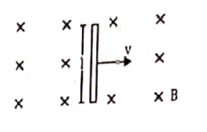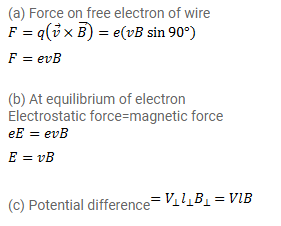# A conducting wire of length l, lying normal to a magnetic field B,

Question:

A conducting wire of length $\mathrm{I}$, lying normal to a magnetic field B, moves a velocity $v$ as shown in figure.

(a) Find the average magnetic force on a free electron of the wire.

(b) Due to this magnetic force, electrons concentrate at one end resulting in an electric field inside the wire. The redistribution stops when the electric force on the free electrons balances the magnetic force on the free electron balances the magnetic force. Find the electric field developed inside the wire when the redistribution stops.

(c) What potential difference is developed between the ends of the wire?Solution: